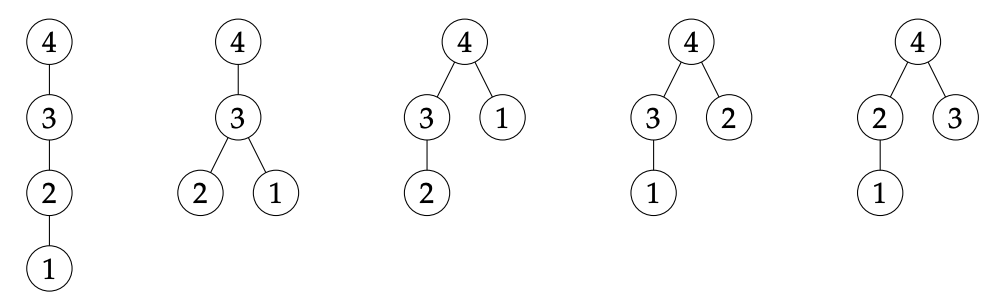시간 제한 메모리 제한 제출 정답 맞은 사람 정답 비율
3 초 512 MB 19 7 7 36.842%

## 문제

The Happy Tree Friends have gathered for their annual meeting, in which they take their most important decisions for the year to come. This year, they will set up a mentoring program to help each other take better care of their loved ones. This programme follows a tree-like hierarchical structure as follows.

The $N$ members of the programme are ranked from $1$ to $N$ (each rank is assigned once), by increasing seniority. For the mentorship programme to be efficient, a person ranked $A$ can mentor a person ranked $B$ only if $A > B$. The most senior Happy Tree Friend can have no mentor, but everybody else has a unique mentor. Conversely, everybody is allowed to mentor from zero to two people.

However, Mr. Pickles, who was assigned the rank $R$, plans to take a sabbatical this year. Thus, he will not be able to mentor anybody, and the Happy Tree Friends should choose their hierarchical structure among those trees in which the node labelled $R$ is a leaf.

Aiming to help his friends to choose such a tree, Mr. Pickles decides to first count how many trees would match his constraint. Unfortunately, he stopped school early, and thus did not learn how to manipulate integers of arbitrary size. Instead, he counts modulo $M$, where $M$ is a fixed positive integer: this is already enough for most purposes in life.

What is the number $L$ that Mr. Pickles will obtain after counting all suitable trees?

## 입력

The input consists of a single line, with three space-separated integers: $R$, $N$, $M$, in that order.

## 출력

The output should contain a single line with the single integer $L$, which is the number of tree-like hierarchical structures that would match Mr. Pickles' constraints, counted modulo $M$.

## 제한

• $1 \leqslant R \leqslant N \leqslant 2021$
• $1 \leqslant M \leqslant 1\,000\,000\,000$

## 예제 입력 1

2 4 2


## 예제 출력 1

1


The node with label $R = 2$ is a leaf in exactly $3$ of five trees listed below, and thus there are $3$ trees that match Mr. Pickles' constraints. The only meaningful feature of our trees is parenthood, which represents mentorship relations, and thus there is no notion of left child or right child of a node. Mr. Pickles counts modulo $M = 2$, and therefore he ends up with the number $L = 3~(\mathrm{mod~}2) = 1$.## 예제 입력 2

2 4 3


## 예제 출력 2

0


Mr. Pickles now counts modulo $M = 3$, and  thus he ends up with the number $L = 3~(\mathrm{mod~}3) = 0$.

## 출처• 문제를 만든 사람: Vincent Jugé